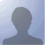If you are looking for TeX support, please go to VietTUG.org

## Problem #943### tim tong cac so sau

Status: New Start Date: 04-12-2011
Priority: Normal Due date:
Assigned to: - % Done:

0%

Category: -
Target version: -

Description

1^2+2^2+3^2+....+n^2

### History

#### #1, #3216Updated by Huu Phuoc Lam [G]over 1 year ago

Vu [G] wrote:

\(1^2+2^2+3^2+....+n^2\)

#### #2, #3217Updated by Nathaniel Goudie [G]over 1 year ago

Vu [G] wrote:

1^2+2^2+3^2+....+n^2

\(1^2+2^2+3^2+....+n^2 \)

/(U_1^2=0)/

#### #4, #3220Updated by Splendid Nguyễn [G]over 1 year ago

hok bik làm :|

#### #5, #3224Updated by -General Poreotix- [G]over 1 year ago

\(U_1^2\)

#### #6, #3226Updated by »—»†•JµsTïÑ•†»—» [G]over 1 year ago

Nathaniel Goudie [G] wrote:

Vu [G] wrote:

1^2+2^2+3^2+....+n^2

/(1^2+2^2+3^2+....+n^2/)

/(1^2+2^2+3^2+....+n^2/)

#### #7, #3227Updated by q [G]over 1 year ago

Vu [G] wrote:

1^2+2^2+3^2+....+n^2

More like AAAAAAAAAAAAAAAAAAAAAAAAAAAAAAAAAAAAAAAAAAAAAAAAAAAAAAAAAAAAAAAAAA

#### #8, #3233Updated by Huu Phuoc Lam [G]over 1 year ago

Huu Phuoc Lam [G] wrote:

Vu [G] wrote:

\(1^2+2^2+3^2+....+n^2\)

\(\Leftrightarrow\)

#### #9, #3236Updated by »—»†•JµsTïÑ•†»—» [G]over 1 year ago

Vu [G] wrote:

1^2+2^2+3^2+....+n^2
Nhức cai đầu >.<!Đề nghị cho bản hướng dẫn

#### #10, #3242Updated by Chloe Chen [G]over 1 year ago

»—»†•JµsTïÑ•†»—» [G] wrote:

Vu [G] wrote:

1^2+2^2+3^2+....+n^2

Nhức cai đầu >.<!Đề nghị cho bản hướng dẫn

Sai chính tả >.

#### #11, #3247Updated by »—»†•JµsTïÑ•†»—» [G]over 1 year ago

Chloe Chen [G] wrote:

»—»†•JµsTïÑ•†»—» [G] wrote:

Vu [G] wrote:

1^2+2^2+3^2+....+n^2

Nhức cai đầu >.<!Đề nghị cho bản hướng dẫn

Sai chính tả >.

Thiếu ti mà lị :D Khó ghê :))

#### #12, #3272Updated by Nguyễn Lê Ngọc Tùng 11C2 [G]over 1 year ago

Vu [G] wrote:

1^2+2^2+3^2+....+n^2

dap an: (n(n+1)(2n+1))/6